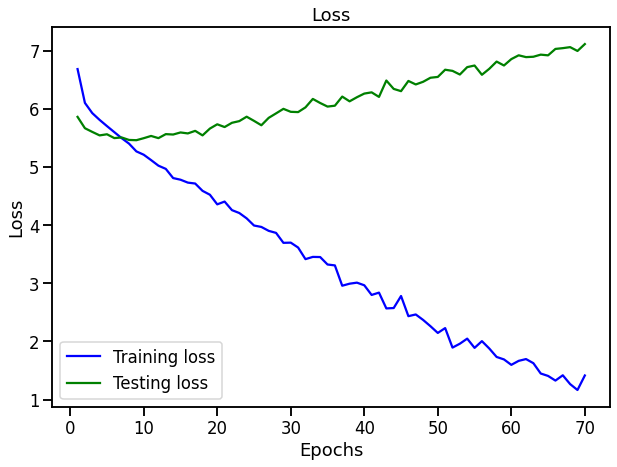# Simple encoder decoder model is overfitting

I have this simple gru based encoder decoder model pretty, its the same model as
seq2seq implementation and yet it is over fitting only after 10 epochs.the translation is english to spanish, without pretrained embeddings for either.

I have also used this model for a different dataset, where both src and trg are english, and i was trying to develop some relation between those, and that overfits after 1 epoch.

I dont know what is the problem, This is bugging me for the past 1 week, any help would highly appreciated.

``````OUTPUT_DIM = eng_vocab_size
INPUT_DIM = esp_vocab_size
ENC_EMB_DIM = 256
DEC_EMB_DIM = 256
HID_DIM = 512
ENC_DROPOUT = 0.5
DEC_DROPOUT = 0.5
enc = Encoder(INPUT_DIM, HID_DIM, ENC_EMB_DIM, ENC_DROPOUT)
dec = Decoder(DEC_EMB_DIM, HID_DIM, OUTPUT_DIM, DEC_DROPOUT)

device = torch.device('cuda' if torch.cuda.is_available() else 'cpu')

model = Seq2Seq(enc, dec, device).to(device)
``````

this is the model initialization.

To counter overfitting, try to add more regularization (dropout, weight decay), decrease the model capacity (smaller or less layers), add augmentation, and make sure the splits are valid (i.e. the data is equally “hard” in both datasets).

1 Like

Apart from @ptrblck’s comment, how large is your dataset? For example, if you use a rather small dataset (maybe just a sample for testing the network), then the distribution of the training and test data could be very different. This would yield in the trends of the losses you observe.

My dataset contains 8000 data points, and the distribution of val set and train set are similar. I also tried with weight decay, but what is happening is the curve doesn’t look over fitting, but something weird is happening. the min loss achieved during overfitting case is almost the same as non overfitting case, and hence i am still getting poor results.